## Circular and Modular Golomb Rulers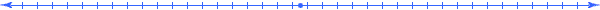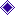IntroductionDefinitionAlgebraic PropertiesWhy Golomb rulers?Perfect Golomb RulersOptimal Golomb Rulers and G(n)A Tighter Lower BoundSearch For Optimal and Near Optimal Golomb RulersModular Golomb RulersJava applet for Golomb RulersLinks to related materialReferences### Introduction

Golomb rulers derive their name from Professor Solomon Golomb who was one of the first to study their construction in relation to combinatorics, coding theory and communications, where most of their applications lie.  Intuitively, a Golomb Ruler may be thought of as a specific type of rulerwhereas a typical ruler has a mark at every unit measure, a Golomb ruler will only have marks at some subset of these measures (see figure 1).

Figure 1. A Golomb ruler of five marks.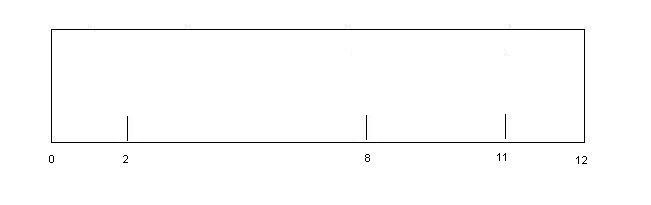Note that the distance between any two marks on the ruler is different than any other two marks.  Any ruler that possesses this trait is called 'Golomb'.### Definition

More formally, we have that a Golomb ruler is a set,

A = {a_1, a_2, ... ,a_N} where a_1<a_2<...<a_N

having the property that the difference,

a_i - a_j (i < j)

is distinct for all pairs (i,j)### Algebraic Properties

Two algebraic transformations under which Golomb rulers are closed are translation and multiplication.

Translation: if A = {a_1, a_2, ... ,a_N} is a Golomb ruler than the set:

A' = {x+a_1, x+ a_2, ... ,x+a_N} is also a Golomb ruler.

proof:  if A is a ruler and A' is not then there must exist some i,j,k,l where,

(x+a_i)+(x+a_j) = (x+a_k)+(x+a_l)

=>  (a_i)+(a_j) = (a_k)+(a_l)

contradicting the fact that A is a Golomb ruler.

Multiplication: if A = {a_1, a_2, ... ,a_N} is a Golomb ruler than the set:

A' = {za_1, z a_2, ... ,za_N}

proof:  if A is a ruler and A' is not then there must exist some i,j,k,l where,

(za_i)+(za_j) = (za_k)+(za_l)

=>  (a_i)+(a_j) = (a_k)+(a_l)

contradicting the fact that A is a Golomb ruler.### Why Golomb Rulers?

Origins

Golomb rulers are credited as being 'discovered' by W. Babcock in 1953.  He was investigating the intermodulation distortion which appeared in third and fifth order consecutive radio bands.  He discovered that by placing each pair of channels inside the frequency spectrum at a distinct distance the third order distortion was eliminated and the fifth order distortion was lessened greatly.

Other Applications

Golomb rulers have a wide variety of other applications, including radio astronomy and information theory.  In radio astronomy astronomers use arrays of radio telescopes in a single line to collect data on particular stars or celestial regions.  More information may be extracted if there are multiple difference measurements between the telescopes.  By placing the telescopes at the marks of a Golomb ruler the number of distance measurements is maximized.

In information theory, Golomb rulers are used for error detection and correction.  Golomb rulers of the same length are used to generate self-orthogonal codes, codes that do not share common differences.  Such codes allow for easier error checking since redundancies between codes will show up as errors and may then be resent or corrected.### Perfect Golomb Rulers

Notice that in the applications above we would like to minimize the amount of space needed to place as many marks as possible.  For example, in Babcock's case, if we have n stations we want to know the minimum bandwidth necessary to fit all n stations in with no interference.  Also, there are certain applications where a ruler measuring every possible distance, while still maintaining the Golomb property, is desired.

Definition:  A Perfect Golomb ruler measures all integer distances from zero to L where L is the length of the ruler.

Figure 2. A perfect Golomb ruler measuring all distances one to six :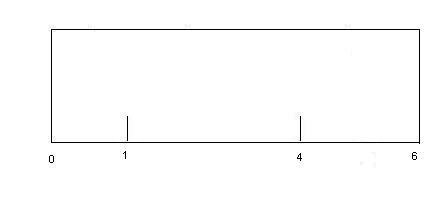A consequence of this definition is that a perfect ruler of n marks is the shortest possible ruler possessing n marks.  This can easily be seen by noting that n marks can measure exactly n choose 2 distances.  For a Golomb ruler with n marks to be perfect it must be of length n choose 2, because if it were shorter then some distance would be measured twice since there are less distances than measurements.  If it were longer, then there must be some lengths that would not be measured between zero and L.  It would seem then that if we wanted to find the smallest ruler with n marks we would simply need to find the corresponding ruler of length n choose 2.  Unfortunately this is not the case.

Theorem: There exist no perfect rulers with more than 4 marks.

Proof:  We will attempt to construct such a ruler.  First, we know that there must be a mark at 0 and a mark at L=(n choose 2), as they are the ends of the ruler.  There must be some set of marks that measure the distance L-1.  There are two possibilities we can measure this distance between 0 and L-1 (0,L-1) or (1,L).  Through  symmetry we know that both are equivalent, so we choose to place a mark at 1.  Now we have to measure the distance L-2.  We can do this in the following three ways: (0,L-2), (1,L-1), (2,L).  The second and third possibilities give us a duplicate measurement of 1, so we place a mark at L-2 to measure the distance.  L-3 is now measured by (1,L-2), so we need to place L-4.  This may be done with (0,L-4), (1,L-3), (2,L-2), (3,L-1), (4,L).  If n=5 then any one of these placements leads to a duplicate entry, with (0,L-4) creating a duplicate entry of 2, (1,L-3) creating a duplicate entry of 1, (2,L-2) creating a duplicate 1, (3,L-1) creating a duplicate 1, (4,L) creating 2 entries of 4 since we have a mark at L-2 = 8 when n=5.  If n>5 then we can place a mark at 4 to make the requirement.  Now we need to place L-5 which can be done with (0,L-5) leading to a duplicate 3, (1, L-4) leading to a duplicate 2, (2,L-3) leading to a duplicate 1, (3,L-2) leading to a duplicate 1, (4, L-1) leading to a duplicate 1 or (5,L) leading to a duplicate 1.  So such a ruler is unable to be constructed.### Optimal Golomb Rulers and G(n)

Unfortunately, the proof above means that we need to abandon perfect rulers as a solution to finding the smallest possible Golomb rulers of n marks.  To reason about this subject, researchers defined optimal Golomb rulers and their related function, G(n).

Definition : An optimal Golomb ruler is a Golomb ruler of shortest possible length dependant on the number of marks.  G(n) denotes this length for a ruler with n marks.

What is interesting about the G(n) function is that we do not know much about it.  In fact, most of the recent research on Golomb rulers has gone into bounding the G(n) function, expanding its known values, as well as finding new, near optimal rulers for larger and larger n.

Some Bounds on G(n):

1. G(n)>=(n/2)(n-1) This was seen in our analysis of the perfect Golomb ruler.

2. G(n)>G(n-1)  In other words, G(n) is a strictly monotonic function.  This is seen by removing the highest mark from the ruler corresponding to G(n).  The resulting ruler will be shorter, and will still be Golomb since we are adding no new distances.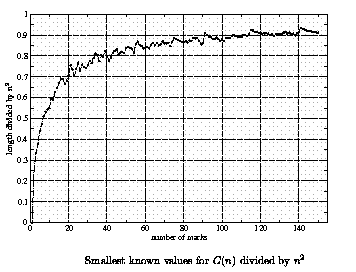An interesting open question is whether or not the following bound holds: G(n)<n^2.  All smallest known values of G(n) are less than n^2 and the figure above points to the function tending towards n^2 as n gets big.### A Tighter Lower Bound

The origin of Golomb rulers extends beyond Babcock and his radio frequencies.  The Golomb ruler was studied extensively by mathematicians for decades before Babcock under the name of Sidon sets.  The Sidon set  problem was first proposed by Simon Sidon in 1932.  Paul Erdos then picked up the problem and published papers on the subject in 1934.  Since then numerous results have been proven.

Definition: A Sidon set is a subset, A = {a_1, a_2,....,a_N} of the natural numbers where i<j => a_i<a_j with the property that the sum of no two elements in the set is equal to the sum of two other elements.  F_2(n) is a function that gives the largest number of integers which can be selected from the first n to form a Sidon set.

Glancing at the definition of Sidon set and F_2(n) we can see that there must be some kind of relation between the two problems.  Indeed, Apostolos Dimitromanolakis proved the equivalence of the two in 2002.

Theorem : A is a Sidon set if A is a Golomb Ruler

proof : Sidon => Golomb

Assume that A is a Sidon set but not a Golomb ruler then there exists i<j<k<m where

a_j-a_i = a_m-a_k => a_j+a_k = a_m+a_i => A is not Sidon.  A contradiction.

Golomb => Sidon

Assume that A is a Golomb ruler but not a Sidon set then there exists i<j<k<m where

a_j+a_k = a_m+a_i => a_j-a_i = a_m-a_k =>A is not Sidon.  A contradiction.

By proving this equivalence, and then a further relation between F_2(n) and G(n,) Dimitromanolakis was able use the pre-existing wealth of knowledge concerning Sidon sets, and apply  it to the Golomb problem, yielding the lower bound that G(n)>n^2-2n*sqrt(n)+sqrt(n)-2.### Search for Optimal and Near Optimal Golomb Rulers

The complexity of finding an optimal Golomb ruler is unknown.  However, it is believed to be NP-Hard and in practice, using the best know methods, finding an optimal ruler of size n+1 has taken approximately ten times the amount of time taken for a ruler of size n.  This huge amount of time has made it so G(n) is only known where n<=24.  This has lead researchers to search for constructions in order to find near optimal Golomb rulers.

Using results from number theory, researches are able to create near optimal rulers in polytime for rulers with a prime number of marks.  The following construction is due to Erdos in 1944 and was discovered in relation to Sidon sets.

A simple Construction for n^2 length ruler: For every prime number p the following sequence forms a Golomb ruler.

2pa+a^2*p, (0<-a<p)

Using this construction and others like it, researchers such as Dimitromanolakis are able to manipulate these efficient constructions for primes to create near optimal rulers for non-prime n.### Modular Golomb Rulers

Modular Golomb Rulers, sometimes called Circular Golomb Rulers, are a particular case of Golomb Rulers that have a near optimal construction.

Definition: A set of k residues a_1, a_2, ... , a_k such that the differences ai-aj (i!=j) are all distinct mod k is called a Modular Golomb Ruler.

Figure 4.  A Modular Golomb Ruler measuring distances 1,2,3,4,6,7: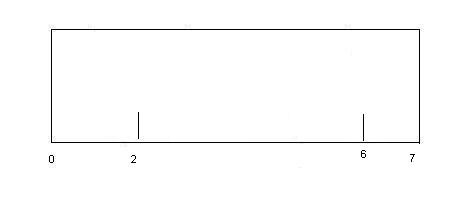Note that this ruler only has 3 marks.  An equivalent way to look at this is as a circle.

Figure 5. The same modular ruler viewed as a circle:Modular Golomb Rulers are equivalent to another mathematical construction called a 'perfect distance set.'  There are many good constructions for perfect distance sets with a prime number of marks.

Some Constructions

Singer: p+1 marks of length p^2+(p+1) 

Bose: p marks of length p^2-1                 

Ruzka: p marks of length p^2-p.             

Another simple counting argument gives us that a ruler with n marks must have n^2-(n+1) distinct distances, making Ruzka's construction near optimal.  Indeed, his construction is used for regular Golomb rulers in an attempt to find near optimal rulers.### Java applet

Link to the Golomb ruler applet.The search for optimal rulers of length 20 and 21

James B. Shearer's Golomb Ruler page with a piece on modular Golomb Rulers

Lloyd Miller's list of best known optimal rulers

The search for the optimal 22 length ruler### References

    W.C. Babcock, Intermodulation interference in radio systems, Bell Systems Technical Journal (1953),     63-73

    R. C. Bose, An affine analogue of Singer's Theorem, J. Ind. Math. Society, 6(1942), p. 1-15

    E.J. Blum, F. Biraud, and J.C. Ribes, On optimal synthetic linear arrays with applications to radioastronomy, IEEE Transactions on Antennas and Propagation 22 (1974), 108-109.

    A Dimitromanolakis, Analysis of the Golomb Ruler and the Sidon Set Problems, and Determination of Large, Near-Optimal Golomb Rulers, Tecnical University of Crete, Department of Electronic and Computer Engineering, 2002.

    P. Erdos and A. Renyi, Additive properties of random sequences of positive integers, Acta Arithmetica 6 (1960), 83-100.

    William T. Rankin, Optimal Golomb rulers: An exhaustive parrell search implementation, Master's thesis, Duke University, department of Electrical Engineering, 1993.

    J. Robinson and A. Bernstein, A class of binary recurrent codes with limited error propagation, IEEE Transactions on Information Theory (1967), 106-113.

    I. Z. Ruzsa, Solving a linear equation in a set of intgers I, Acta Arithmetica, 65(1993), p. 259-282.

    J. Singer, A theorem in finite projective geometry and some applications to number theory, Transactions American Mathematical Society, 43(1938), p. 377-385.